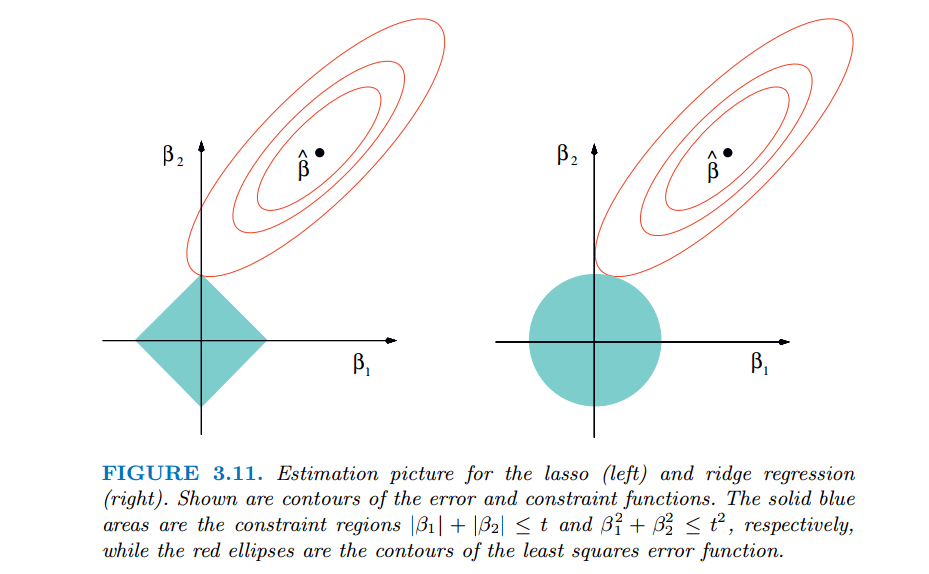# Linear regression

• 对于n维特征的样本数据，使用线性回归模型：
$h_\theta(x_1, x_2, ...x_n) = \theta_0 + \theta_{1}x_1 + ... + \theta_{n}x_{n}$

其中𝜃𝑖 (i = 0,1,2… n)为模型参数，𝑥𝑖 (i = 0,1,2… n)为每个样本的n个特征值。

• 我们增加一个特征𝑥0=1：
$h_\theta(x_0, x_1, ...x_n) = \sum\limits_{i=0}^{n}\theta_{i}x_{i}$
线性回归用均方误差作为损失函数：
$J(\theta_0, \theta_1..., \theta_n) = \sum\limits_{i=1}^{m}(h_\theta(x_0^{(i)}, x_1^{(i)}, ...x_n^{(i)}) - y_i)^2$
矩阵表示：$J(\mathbf\theta) = \frac{1}{2}(\mathbf{X\theta} - \mathbf{Y})^T(\mathbf{X\theta} - \mathbf{Y})$

1. 梯度下降法，则𝜃的迭代公式：$\mathbf\theta= \mathbf\theta - \alpha\mathbf{X}^T(\mathbf{X\theta} - \mathbf{Y})$

2. 最小二乘法，则𝜃的结果公式：$\mathbf{\theta} = (\mathbf{X^{T}X})^{-1}\mathbf{X^{T}Y}$

• 如果使用线性回归模型：
$h_\theta(x_1, x_2) = \theta_0 + \theta_{1}x_1 + \theta_{2}x_{2} + \theta_{3}x_1^{2} + \theta_{4}x_2^{2} + \theta_{5}x_{1}x_2$

• 我们令$x_0 = 1, x_1 = x_1, x_2 = x_2, x_3 =x_1^{2}, x_4 = x_2^{2}, x_5 = x_{1}x_2$，对于每个二元样本特征(𝑥1,𝑥2)，得到一个五元样本特征(1,𝑥1,𝑥2,𝑥21,𝑥22,𝑥1𝑥2)，通过这个改进的五元样本特征，重新把不是线性回归的函数变回线性回归。

• 模型函数：$ln\mathbf{Y} = \mathbf{X\theta}$

• 我们将Y一般化：$\mathbf{g}(\mathbf{Y}) = \mathbf{X\theta}$

重新回到线性模型。

• 为了防止模型的过拟合，在建立线性模型的时候加入正则化项。

L1正则化

• 线性回归的L1正则化通常称为Lasso回归，常数系数𝛼调节损失函数的均方差和正则化项的权重，具体Lasso回归的损失函数表达式如下：
$J(\mathbf\theta) = \frac{1}{2}(\mathbf{X\theta} - \mathbf{Y})^T(\mathbf{X\theta} - \mathbf{Y}) + \alpha||\theta||_1$

• Lasso回归的求解办法一般有坐标轴下降法（coordinate descent）和最小角回归法（ Least Angle Regression）

• Lasso回归可以使得一些特征的系数变小，甚至一些绝对值较小的系数直接变为0。增强模型的泛化能力。

L2正则化

• 线性回归的L2正则化称为Ridge回归，具体Ridge回归的损失函数表达式如下：
$J(\mathbf\theta) = \frac{1}{2}(\mathbf{X\theta} - \mathbf{Y})^T(\mathbf{X\theta} - \mathbf{Y}) + \frac{1}{2}\alpha||\theta||_2^2$

• Ridge回归的求解一般用最小二乘法。

令𝐽(𝜃)的导数为0：$\mathbf{X^T(X\theta - Y) + \alpha\theta} = 0$
求得：
$\mathbf{\theta = (X^TX + \alpha E)^{-1}X^TY}$
其中E为单位矩阵

• Lasso是以缩小变量集（降维）为思想的压缩估计方法。它通过将变量的系数进行压缩并使某些回归系数变为0，进而达到变量选择的目的。
• L1$\Rightarrow$ 参数稀疏$\Rightarrow$ 特征选择(Feature Selection)，可解释性(Interpretability)
• L2$\Rightarrow$ 参数变小$\Rightarrow$ 模型变简单$\Rightarrow$ 优化求解变得稳定和快速• 红色的椭圆代表随着𝛼 的变化所得到的残差平方和，βˆ为椭圆的中心点，对应普通线性模型的最小二乘估计，等高线与约束域首次相交的地方就是最优解：
• Ridge其切点只会存在于圆周上，相切不会在坐标轴上，则在任一维度的取值都不为0，因此没有稀疏；Lasso会存在切点在坐标轴上的情况，使得部分维度特征权重为0，因此很容易产生稀疏的结果。
• L1会趋向于产生少量的特征，其他的特征都是0，而L2会选择更多的特征，这些特征都接近于0。L2提高精度且较好适应、拟合。L1有利于稀疏数据特征。
• 理论上，L1+L2=Elastic Nets，既可以处理稀疏问题，同时也可以保证精度。
但是实际上引入超参数会难以适当，成本较高，很少有L1+L2的效果优于L2的

©️2019 CSDN 皮肤主题: 游动-白 设计师: 上身试试